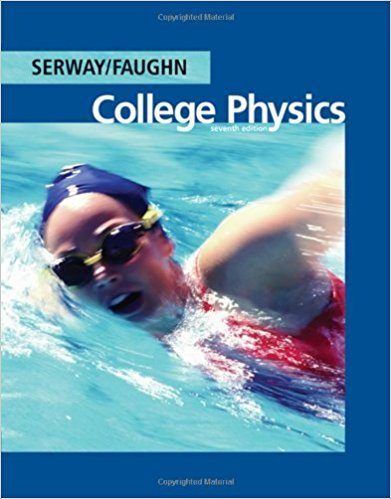×
Get Full Access to College Physics - 7 Edition - Chapter 18 - Problem 18.36
Get Full Access to College Physics - 7 Edition - Chapter 18 - Problem 18.36

×

# A series RC circuit has a time constant of 0.960 s. TheISBN: 9780495113690 154

## Solution for problem 18.36 Chapter 18

College Physics | 7th Edition

• Textbook Solutions
• 2901 Step-by-step solutions solved by professors and subject experts
• Get 24/7 help from StudySoup virtual teaching assistantsCollege Physics | 7th Edition

4 5 1 396 Reviews
18
4
Problem 18.36

A series RC circuit has a time constant of 0.960 s. The battery has an emf of 48.0 V, and the maximum current in the circuit is 0.500 mA. What are (a) the value of the capacitance and (b) the charge stored in the capacitor 1.92 s after the switch is closed?

Step-by-Step Solution:
Step 1 of 3

Lectures 8 nd Dynamics ­ KINEMATICS describes “how” things move, DYNAMICS describes “why” things move ­ Unbalanced Force causes motion to c hange (Δv ) Force → a physical uantity escribing how hard and in what direction acceleration is moving (push or pull) ­ Requires an agent a nd object ­ Is a vector Contact Force ­ Physically touches o bject ­ The agent is exactly what is in contact with the object Long Range Force ­ Not touching (gravity is the only one

Step 2 of 3

Step 3 of 3

##### ISBN: 9780495113690

This textbook survival guide was created for the textbook: College Physics, edition: 7. Since the solution to 18.36 from 18 chapter was answered, more than 256 students have viewed the full step-by-step answer. College Physics was written by and is associated to the ISBN: 9780495113690. The answer to “A series RC circuit has a time constant of 0.960 s. The battery has an emf of 48.0 V, and the maximum current in the circuit is 0.500 mA. What are (a) the value of the capacitance and (b) the charge stored in the capacitor 1.92 s after the switch is closed?” is broken down into a number of easy to follow steps, and 52 words. The full step-by-step solution to problem: 18.36 from chapter: 18 was answered by , our top Physics solution expert on 11/15/17, 04:28PM. This full solution covers the following key subjects: Circuit, constant, capacitor, Charge, Battery. This expansive textbook survival guide covers 30 chapters, and 2135 solutions.

Unlock Textbook Solution